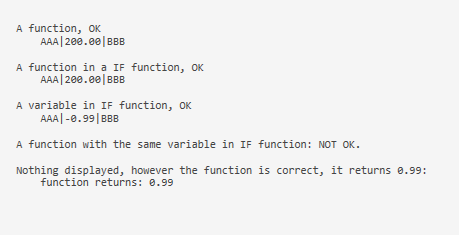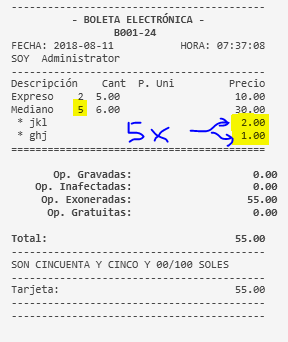# Print Template IF [] with calcaluation [=F(xxx)]

Hi,

It looks like that putting twice the [ … [ … ] …] give me problem.

``````[LAYOUT]

{ORDERS}

[ORDERS]

VL00VNIU|{QUANTITY}|CODE|-|{NAME}|{PRICE}|0|9997|0|EXO|VAT|E|20|0.0|-|0.00|||||0|{PRICE}|{TOTAL PRICE}|0|-|0|0|0|0|0|0|0
{ORDER TAGS}

[ORDER TAGS]
-- Format for order tags
[='{ORDER TAG PRICE}' != 0 ? 'NIU|{QUANTITY}|CODE|-|{ORDER TAG NAME}|{ORDER TAG PRICE}|0|9997|0|EXO|VAT|E|20|0.0|-|0.00|||||0|{ORDER TAG PRICE}|[=F(TN('{QUANTITY}') * TN('{ORDER TAG PRICE}'))]|0|-|0|0|0|0|0|0|0' : '']
``````

When I put

``````|{QUANTITY}{ORDER TAG PRICE}|
``````

it shows, e.g. : 2*25 (normal)

when I put the formula, nothing is displayed.

``````[=F(TN('{QUANTITY}') * TN('{ORDER TAG PRICE}'))]
``````

Is it because the formula is inside the IF[]?

Marc

IIs nothing being displayed when an order is added without an order tag?

This will be because adding square brackets around a line in the ticket template stops that line showing on the print out if it’s value is zero

[=F(TN(’{QUANTITY}’) * TN(’{ORDER TAG PRICE}’))]

If there is no order tag then there’s is no order tag price so that value becomes zero, so when quantity is multiplied by zero the result is zero. So the square brackets then act to hide that line from the ticket print out as

On your template is the line that has the square brackets at the start and end coloured in black? If so this is the reason it doesn’t show as those square brackets serve to only show a value on the ticket printout if the value is greater than zero

Might be useful to show that part of your ticket template

There are values …

Here a small template to illustrate what I mean:

``````[LAYOUT]
<L00>A function, OK
<L00>    AAA|[=F(100 + 100)]|BBB
<L00>
<L00>A function in a IF function, OK
[= 0 == 0 ? '<L00>    AAA|[=F(100 + 100)]|BBB' : '']
<L00>
<L00>A variable in IF function, OK
[= 0 == 0 ? '<L00>    AAA|{CALCULATION TOTAL:Discount}|BBB' : '']
<L00>
<L00>A function with the same variable in IF function: NOT OK.
[= 0 == 0 ? '<L00>AAA|[=F(-1 * TN('{CALCULATION TOTAL:Discount}'))]|BBB' : '']
<L00>
<L00>Nothing displayed, however the function is correct, it returns 0.99:
<L00>    function returns: [=F(-1 * TN('{CALCULATION TOTAL:Discount}'))]
``````

Result:You can see that in 2. there are brackets [=F(100 + 100)] and it works
In 4. brackets (function) + variable and it returns nothing

This is not a valid string so ternary operator won’t work properly.

``````0 == 0 ? '<L00>AAA|[=F(-1 * TN('{CALCULATION TOTAL:Discount}'))]|BBB' : ''
``````

In fact it won’t work even you’ll be able to fix that because nesting expressions like `[=x[=x]]` is not supported.

I got the same problem to print the DETAILED ORDER file (not paper print):

``````IF ORDER TAG has no price
THEN don't print the TAG line
ELSE print the tag line
``````

which is:

`[='{ORDER TAG PRICE}' != 0 ? 'Print the line' : '']`

So one [] (brackets expression)

Now comes my issue.
If I have 2 ORDER TAGS with price and a multiple order (5 mediano), like below:I need to have the total of each ORDER TAG line and:

• I cannot use ORDER TAG PRICE, as it is the price of one ORDER TAG.
• I cannot use ORDER TAG TOTAL, as it is the total price of BOTH order tags. (15)
• I cannot use {Quantity}X{Order Tag Price}, as it would be nesting expressions like `[=x[=x]]` which is not possible.

Can I use a script?

If yes, as I don’t have the DB layout, can somebody help me with the SQL to

``````SELECT [Order Quantity]  X [Order Tag Price]) as [Order_Tag_Total_Price_Per_Tag]
WHERE ??? ORDER = the current ORDER and ORDER TAG = the current ORDER TAG
``````

I have no clue on how to pass that information to the script/function in the [ORDER TAGS] section using {Order TAGS}, I don’t know if this is possible neigther

I am not trying to make things difficult, I am live and that scenario happened yesterday and the electronic bill was rejected by the tax administration system because the sum of the lines did not match the total; I was using ORDER TAG TOTAL

So
TAG jkl shows total tag price 15 instead of 10
TAG ghj shows total tag price 15 instead of 5

Marc

OK. I added a new tag for next update so you’ll be able to configure order tag line as …

``````[ORDER TAGS]
-- Format for order tags
<J00> * {ORDER TAG NAME} | [={QUANTITY}>1 && {ORDER TAG PRICE} > 0?'({QUANTITY} x {ORDER TAG PRICE})':''] [{ORDER TAG TOTAL:{ORDER TAG GROUP}={ORDER TAG NAME}}]
``````

This will print order tag total and also a breakdown of the price if order quantity is greater than one. If order quantity is 1 it will print just the price.

2 Likes

Thank you very much emre.
Can you tell me more or less the period of issuing an update?

In the mean time, I’ll tell the waitress not to submit multiple orders with tags.

I added your account to beta group so you’ll better track the updates. When it is released you’ll see it on the release notes section. Generally we update beta versions multiple times in a week. We release it to public when all new features tested.

Could finally make it that way, however I am waiting for emre solution in the new release.

`[='{ORDER TAG PRICE}' != 0 ? 'Sone fields' + Number({QUANTITY} * {ORDER TAG PRICE}).toFixed(2) + 'some fields' : '']`

`+ {QUANTITY} * {ORDER TAG PRICE} +`

Works also but gives me some error due to the number format, I had:
3 x 2.70 = 8.1000000001 and [=FF()] cannot be use, so:

`+ Number({QUANTITY} * {ORDER TAG PRICE}).toFixed(2) +`

gives 8.10

Hello everyone, I have read all the messages in this post but I can not understand.

It is possible to print only order tag name only if it has a price and if it has no price do not print?

Thank you

Yes just put square brackets around it […] the square brackets will only show whats inbetween them on the receipt if it has a value

so for example in my template a gin and tonic has the tonic as an order tag, as you can see this part is between square brackets, so it only prints on the receipt if an item with an tonic order tag is added to the receipt

[<div style=“font-size:13px;text-align:left;font-family:‘Calibri’”>
<table>
<tr>
<td width=“196”> - {ORDER TAG:Tonic}</td>
<td width=45 align=right></td>
</tr>
</table>
</div>]

Thanks for your answer, I’m sorry but with so much code I do not understand.
This is my ticket template:

ticket template.pdf (10.8 KB)

``````[LAYOUT]
-- General layout
<C00>VENTA PULA
<C00>CIF: 27336502-J
<C00>TELF: 952 11 30 68
<F>*
<L00>Fecha:{TICKET DATE}
<L00>Hora:{TIME}
<F>-
{ENTITIES}

<F>-
{ORDERS}
<F>=
<EB>
{DISCOUNTS}
[<J10>Total Regalo:|{ORDER STATE TOTAL:Regalo}]
{SERVICES}
<J10>Total(iva incluido):|{TICKET TOTAL}
<F>-
{PAYMENTS}
<DB>
<F>=
<C10>GRACIAS POR SU VISITA

[DISCOUNTS]
<J00>{CALCULATION NAME} %{CALCULATION AMOUNT}|{CALCULATION TOTAL}

[SERVICES]
<J00>{CALCULATION NAME}|{CALCULATION TOTAL}

[PAYMENTS]
<J00>Cambio:|{CHANGE TOTAL}
<XCT>27,112,0,25,250

[ORDERS]
-- Default format for orders
<J00>- {QUANTITY} {NAME}|{TOTAL PRICE}
{ORDER TAGS}

[ENTITIES:Mesa]
-- Table entity format
<L00>Mesa: {ENTITY NAME}

[ENTITIES:Cliente]
-- Customer entity format
<J00>Cliente: {ENTITY NAME}
<L00>CIF:{ENTITY DATA:DNI}
<L00>DIRECCION:
<L00>{ENTITY DATA:Dirección}

[ORDER TAGS]
-- Default format for orders
<J00> * {ORDER TAG NAME} |{ORDER TAG PRICE}
``````

I changed the last line for this one:

``````<J00> [* {ORDER TAG NAME} | [={QUANTITY}>1 && {ORDER TAG PRICE} > 0?'({QUANTITY} x {ORDER TAG PRICE})':''] [{ORDER TAG TOTAL:{ORDER TAG GROUP}={ORDER TAG NAME}}]]
``````

Can you show me the error?

I simply copied myself.

Sorry and thanks

Yes that is wrong you can’t imbed multiple brackets like that.

What is that supposed to do?

Try this:

``````[ORDER TAGS]
-- Format for order tags
[<J00>    - {ORDER TAG NAME} | {ORDER TAG PRICE}]
``````

Look at it now I edited it I gave you wrong thing to try.

EDIT: Ugh ok now its right one I kept copying wrong one sorry. Try whats there now.

1 LikeWOW, I just copied what I said in the previous answers as a solution, and in the end it was as simple as putting square brackets?
It works but I do not understand it.

Thank you very much for your answer, but you can explain it to me.

There is not much to explain. It was designed to work that way. Its just a trick we can use.

Basically the brackets force it to only show a string if it has a value.